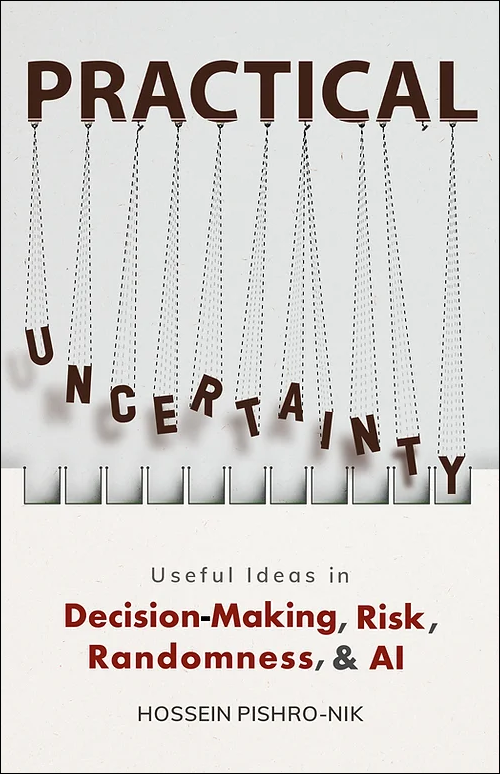## 7.1.1 Law of Large Numbers

The law of large numbers has a very central role in probability and statistics. It states that if you repeat an experiment independently a large number of times and average the result, what you obtain should be close to the expected value. There are two main versions of the law of large numbers. They are called the weak and strong laws of the large numbers. The difference between them is mostly theoretical. In this section, we state and prove the weak law of large numbers (WLLN). The strong law of large numbers is discussed in Section 7.2. Before discussing the WLLN, let us define the sample mean.

Definition . For i.i.d. random variables $X_1, X_2 , ... , X_{\large n}$, the sample mean, denoted by $\overline{X}$, is defined as \begin{align}%\label{} \overline{X}=\frac{X_1+X_2+...+X_{\large n}}{n}. \end{align} Another common notation for the sample mean is $M_{\large n}$. If the $X_i$'s have CDF $F_X(x)$, we might show the sample mean by $M_{\large n}(X)$ to indicate the distribution of the $X_{\large i}$'s.

Note that since the $X_{\large i}$'s are random variables, the sample mean, $\overline{X}=M_{\large n}(X)$, is also a random variable. In particular, we have \begin{align}%\label{} E[\overline{X}]&=\frac{EX_1+EX_2+...+EX_{\large n}}{n} &\textrm{(by linearity of expectation)}\\ &=\frac{nEX}{n} &\textrm{(since $EX_{\large i}=EX$)}\\ &=EX. \end{align} Also, the variance of $\overline{X}$ is given by \begin{align}%\label{} \mathrm{Var}(\overline{X})&=\frac{\mathrm{Var}(X_1+X_2+...+X_{\large n})}{n^2} &\textrm{(since $\mathrm{Var}(aX)=a^2\mathrm{Var}(X)$)}\\ &=\frac{\mathrm{Var}(X_1)+\mathrm{Var}(X_2)+...+\mathrm{Var}(X_{\large n})}{n^2} &\textrm{(since the $X_{\large i}$'s are independent)}\\ &=\frac{n\mathrm{Var}(X)}{n^2} &\textrm{(since $\mathrm{Var}(X_{\large i})=\mathrm{Var}(X)$)}\\ &=\frac{\mathrm{Var}(X)}{n}. \end{align}

Now let us state and prove the weak law of large numbers (WLLN).

The weak law of large numbers (WLLN)

Let $X_1$, $X_2$ , ... , $X_{\large n}$ be i.i.d. random variables with a finite expected value $EX_{\large i}=\mu < \infty$. Then, for any $\epsilon>0$, \begin{align}%\label{} \lim_{n \rightarrow \infty} P(|\overline{X}-\mu| \geq \epsilon)=0. \end{align}

• Proof
• The proof of the weak law of large number is easier if we assume $\mathrm{Var}(X)=\sigma^2$ is finite. In this case we can use Chebyshev's inequality to write \begin{align}%\label{} P(|\overline{X}-\mu| \geq \epsilon) &\leq \frac{\mathrm{Var}(\overline{X})}{\epsilon^2}\\ =\frac{\mathrm{Var}(X)}{n \epsilon^2}, \end{align}

which goes to zero as $n \rightarrow \infty$.

 The print version of the book is available on Amazon.Practical uncertainty: Userful Ideas in Decision-Making, Risk, Randomness, & AI